# RD Sharma Solutions for Class 10 Maths Chapter 3 Pair of Linear Equations in Two Variables Exercise 3.8

Application to problems based on fractions is an interesting one. The RD Sharma Solutions Class 10 can help students rectify any conceptual doubts regarding any chapter in Class 10 Maths. All the solutions are created by expert faculty at BYJU’S, which is on par with the latest CBSE marking schemes. For this exercise, the RD Sharma Solutions for Class 10 Maths Chapter 3 Pair of Linear Equations in Two Variables Exercise 3.8 PDF is available for download below.

## RD Sharma Solutions for Class 10 Maths Chapter 3 Pair of Linear Equations in Two Variables Exercise 3.8 Download PDF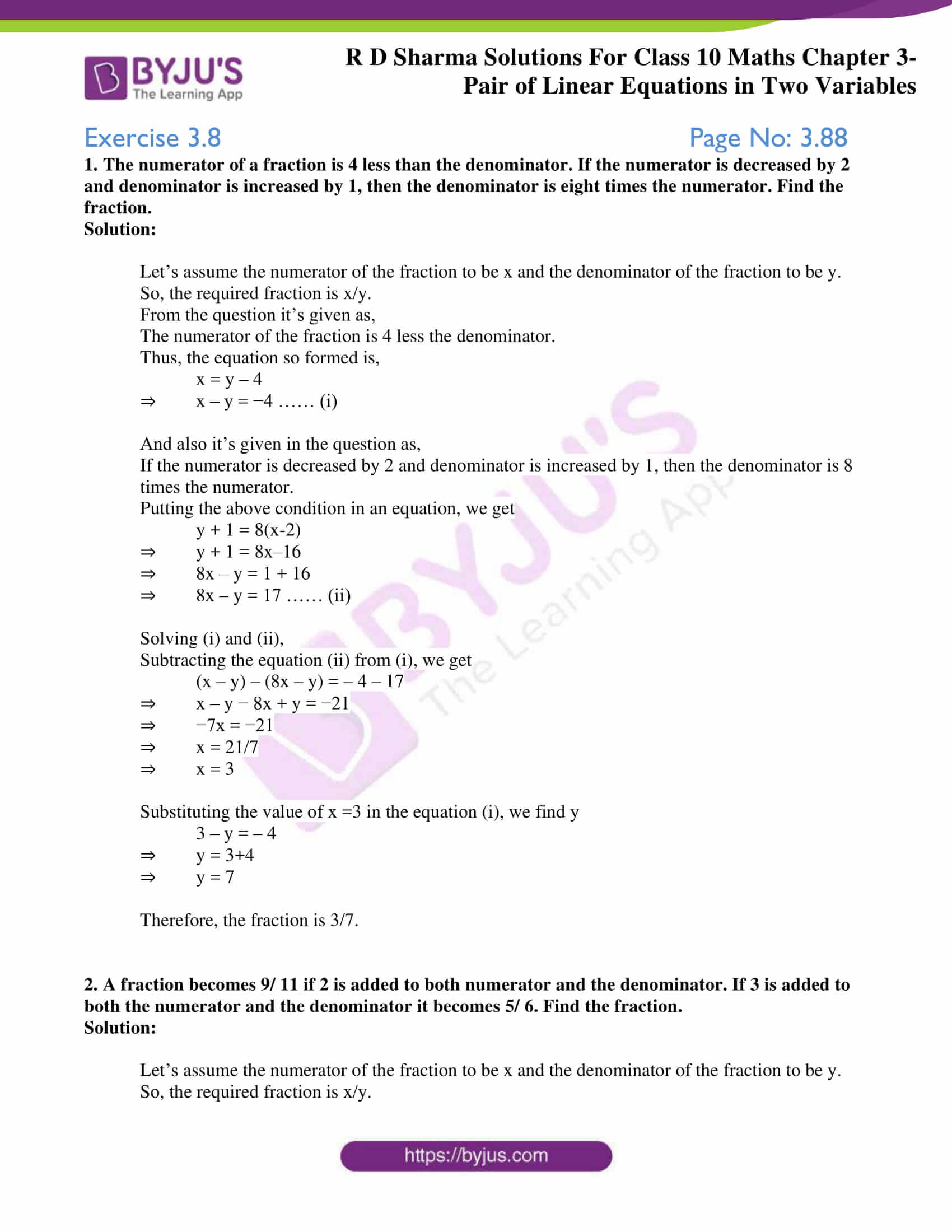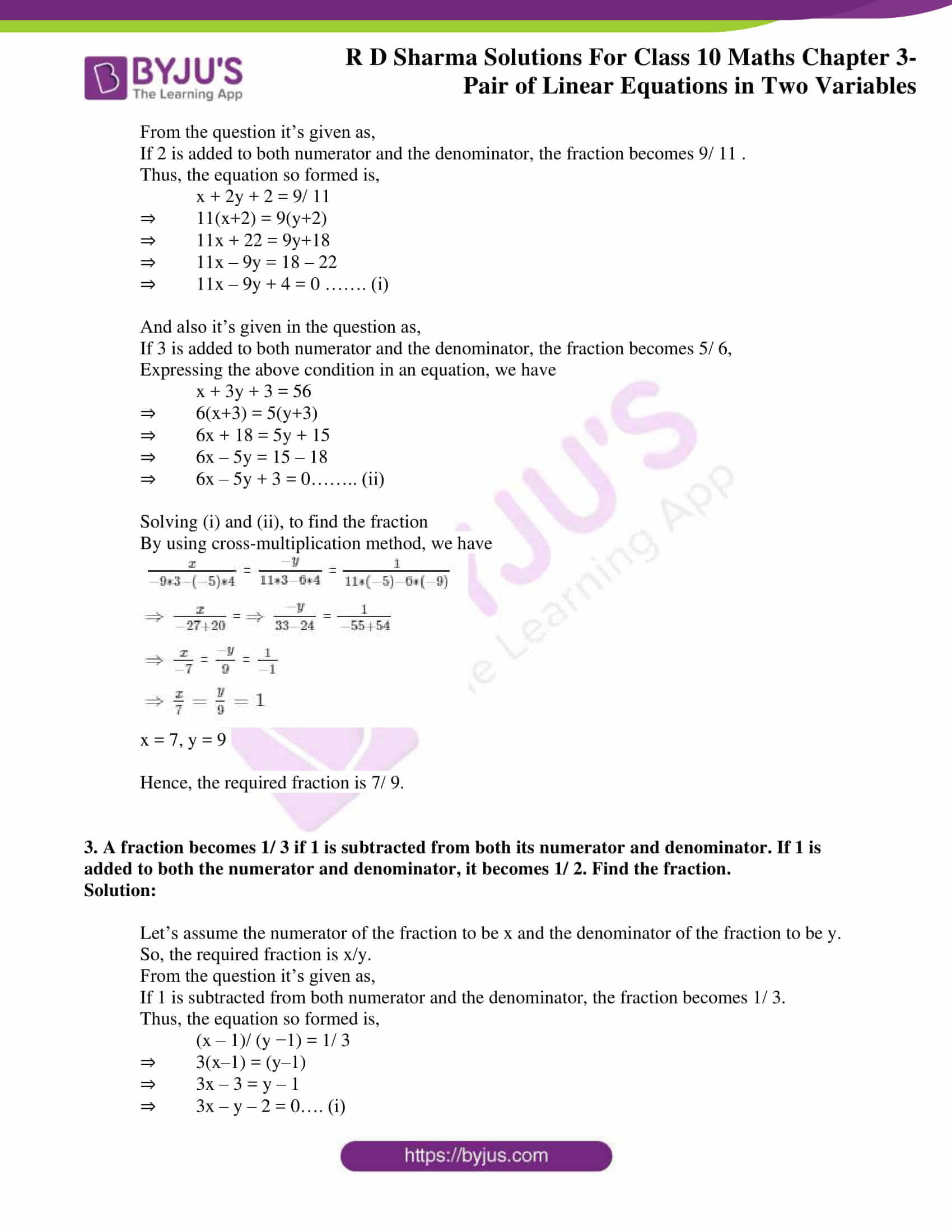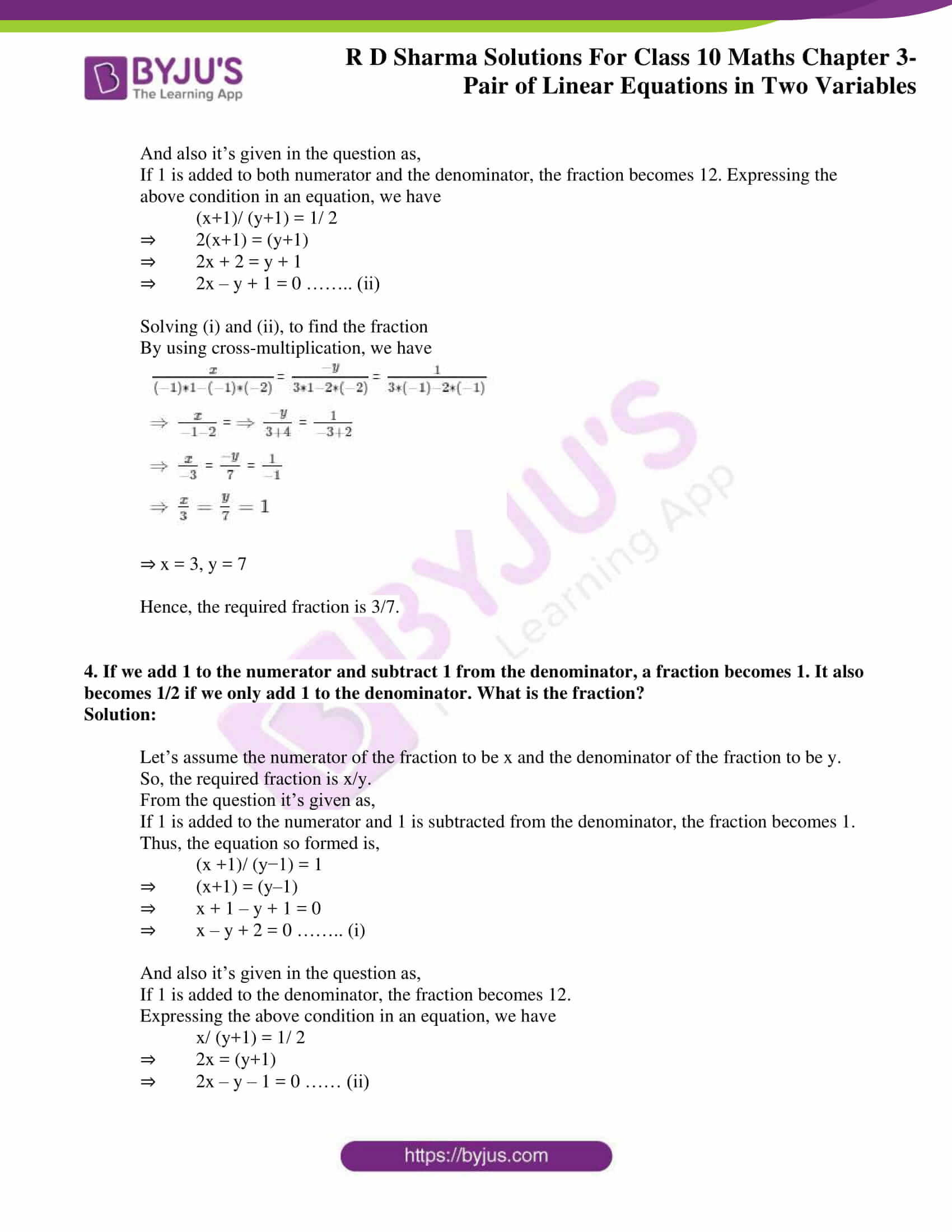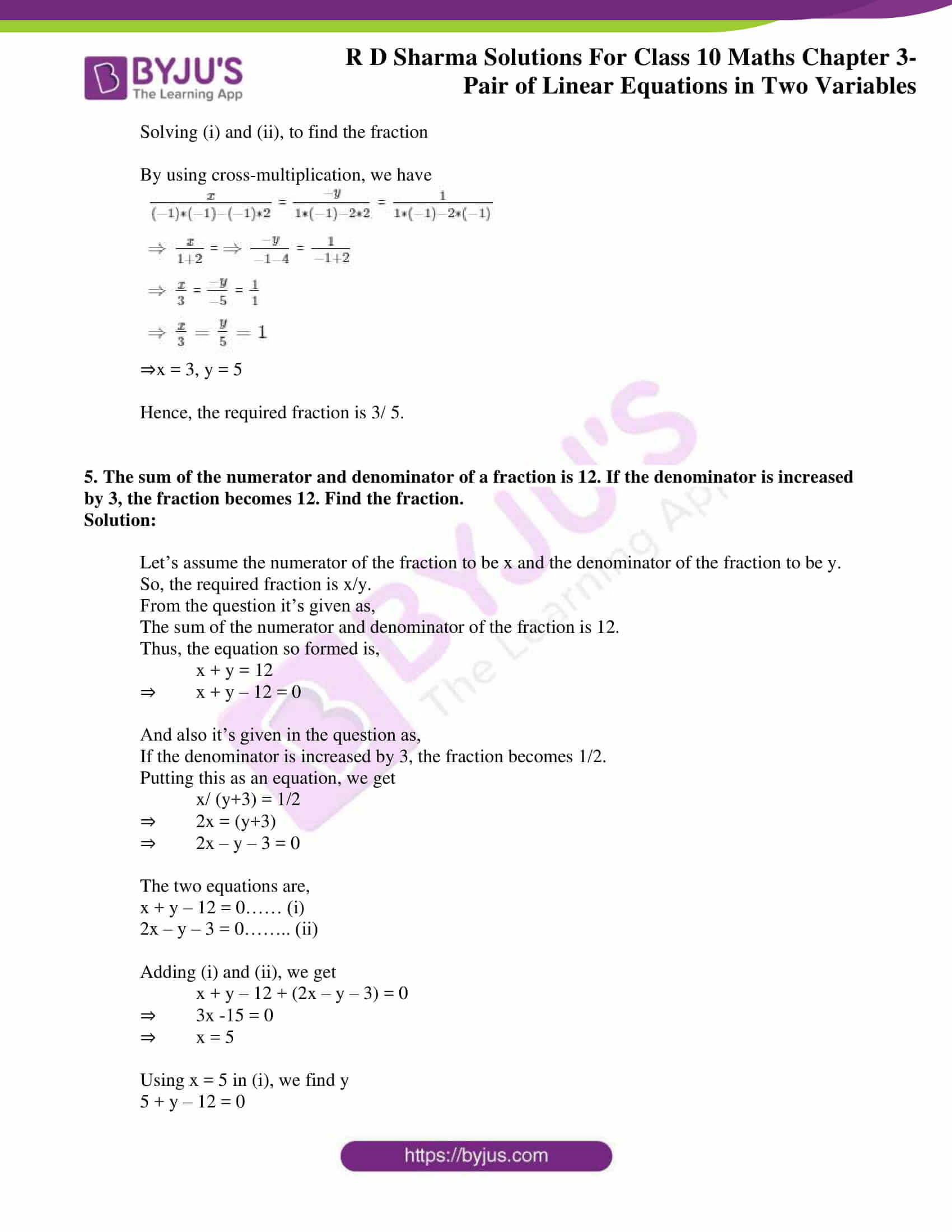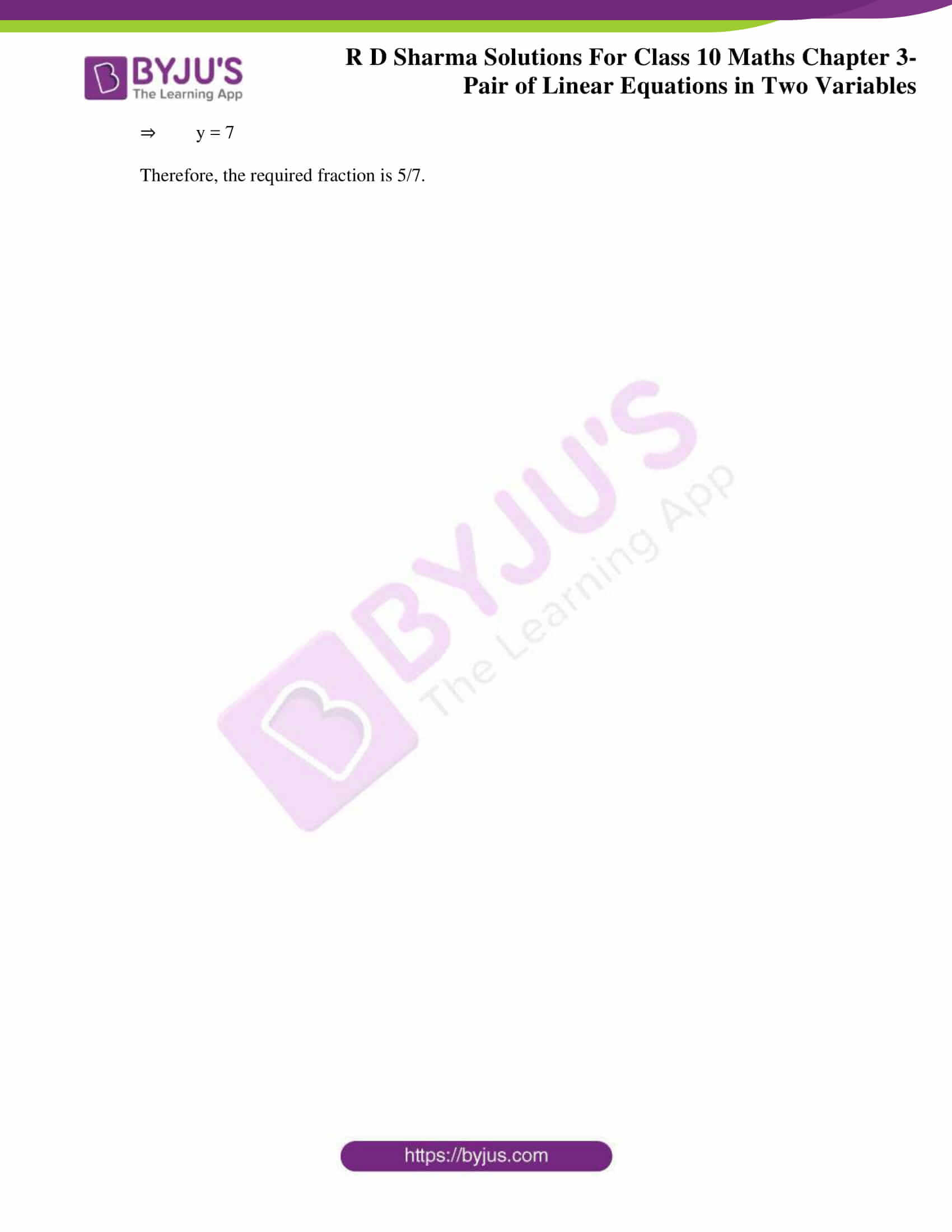### Access RD Sharma Solutions for Class 10 Maths Chapter 3 Pair of Linear Equations In Two Variables Exercise 3.8

1. The numerator of a fraction is 4 less than the denominator. If the numerator is decreased by 2 and denominator is increased by 1, then the denominator is eight times the numerator. Find the fraction.

Solution:

Let’s assume the numerator of the fraction to be x and the denominator of the fraction to be y.

So, the required fraction is x/y.

From the question, it’s given that:

The numerator of the fraction is 4 less than the denominator.

Thus, the equation so formed is,

x = y – 4

⇒ x – y = −4 …… (i)

And also, it’s given in the question as,

If the numerator is decreased by 2 and the denominator is increased by 1, then the denominator is 8 times the numerator.

Putting the above condition in an equation, we get

y + 1 = 8(x-2)

⇒ y + 1 = 8x–16

⇒ 8x – y = 1 + 16

⇒ 8x – y = 17 …… (ii)

Solving (i) and (ii),

Subtracting equation (ii) from (i), we get

(x – y) – (8x – y) = – 4 – 17

⇒ x – y − 8x + y = −21

⇒ −7x = −21

⇒ x = 21/7

⇒ x = 3

Substituting the value of x =3 in equation (i), we find y

3 – y = – 4

⇒ y = 3+4

⇒ y = 7

Therefore, the fraction is 3/7.

2. A fraction becomes 9/ 11 if 2 is added to both numerator and the denominator. If 3 is added to both the numerator and the denominator it becomes 5/ 6. Find the fraction.

Solution:

Let’s assume the numerator of the fraction to be x and the denominator of the fraction to be y.

So, the required fraction is x/y.

From the question, it’s given as,

If 2 is added to both the numerator and the denominator, the fraction becomes 9/ 11 .

Thus, the equation so formed is,

x + 2y + 2 = 9/ 11

⇒ 11(x+2) = 9(y+2)

⇒ 11x + 22 = 9y+18

⇒ 11x – 9y = 18 – 22

⇒ 11x – 9y + 4 = 0 ……. (i)

And also, it’s given in the question as,

If 3 is added to both the numerator and the denominator, the fraction becomes 5/ 6,

Expressing the above condition in an equation, we have

x + 3y + 3 = 56

⇒ 6(x+3) = 5(y+3)

⇒ 6x + 18 = 5y + 15

⇒ 6x – 5y = 15 – 18

⇒ 6x – 5y + 3 = 0…….. (ii)

Solving (i) and (ii), to find the fraction

By using the cross-multiplication method, we have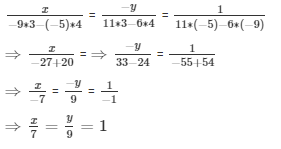x = 7, y = 9

Hence, the required fraction is 7/ 9.

3. A fraction becomes 1/ 3 if 1 is subtracted from both its numerator and denominator. If 1 is added to both the numerator and denominator, it becomes 1/ 2. Find the fraction.

Solution:

Let’s assume the numerator of the fraction to be x and the denominator of the fraction to be y.

So, the required fraction is x/y.

From the question, it’s given that:

If 1 is subtracted from both the numerator and the denominator, the fraction becomes 1/ 3.

Thus, the equation so formed is,

(x – 1)/ (y −1) = 1/ 3

⇒ 3(x–1) = (y–1)

⇒ 3x – 3 = y – 1

⇒ 3x – y – 2 = 0…. (i)

And also, it’s given in the question as,

If 1 is added to both the numerator and the denominator, the fraction becomes 12. Expressing the above condition in an equation, we have

(x+1)/ (y+1) = 1/ 2

⇒ 2(x+1) = (y+1)

⇒ 2x + 2 = y + 1

⇒ 2x – y + 1 = 0 …….. (ii)

Solving (i) and (ii), to find the fraction

By using cross-multiplication, we have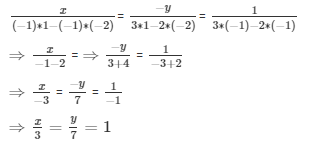⇒ x = 3, y = 7

Hence, the required fraction is 3/7.

4. If we add 1 to the numerator and subtract 1 from the denominator, a fraction becomes 1. It also becomes 1/2 if we only add 1 to the denominator. What is the fraction?

Solution:

Let’s assume the numerator of the fraction to be x and the denominator of the fraction to be y.

So, the required fraction is x/y.

From the question, it’s given that:

If 1 is added to the numerator and 1 is subtracted from the denominator, the fraction becomes 1.

Thus, the equation so formed is,

(x +1)/ (y−1) = 1

⇒ (x+1) = (y–1)

⇒ x + 1 – y + 1 = 0

⇒ x – y + 2 = 0 …….. (i)

And also, it’s given in the question as,

If 1 is added to the denominator, the fraction becomes 12.

Expressing the above condition in an equation, we have

x/ (y+1) = 1/ 2

⇒ 2x = (y+1)

⇒ 2x – y – 1 = 0 …… (ii)

Solving (i) and (ii), to find the fraction

By using cross-multiplication, we have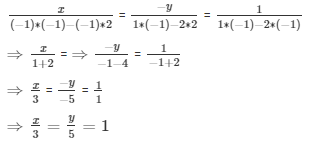⇒x = 3, y = 5

Hence, the required fraction is 3/ 5.

5. The sum of the numerator and denominator of a fraction is 12. If the denominator is increased by 3, the fraction becomes 12. Find the fraction.

Solution:

Let’s assume the numerator of the fraction to be x and the denominator of the fraction to be y.

So, the required fraction is x/y.

From the question, it’s given that:

The sum of the numerator and denominator of the fraction is 12.

Thus, the equation so formed is,

x + y = 12

⇒ x + y – 12 = 0

And also, it’s given in the question as,

If the denominator is increased by 3, the fraction becomes 1/2.

Putting this as an equation, we get

x/ (y+3) = 1/2

⇒ 2x = (y+3)

⇒ 2x – y – 3 = 0

The two equations are,

x + y – 12 = 0…… (i)

2x – y – 3 = 0…….. (ii)

Adding (i) and (ii), we get

x + y – 12 + (2x – y – 3) = 0

⇒ 3x -15 = 0

⇒ x = 5

Using x = 5 in (i), we find y

5 + y – 12 = 0

⇒ y = 7

Therefore, the required fraction is 5/7.